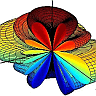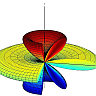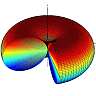One of the surprising consequences of the electromagnetic theory is that electromagnetic waves can carry energy even through vacuum and without the need of any medium. This property of electromagnetic waves is at the core of countless applications in broadcasting, mobile communications, remote sensing, and so forth.

To make all of these technologies possible we need some devices to radiate and receive electromagnetic waves. Such devices are generally called antennas. Antennas are essential components in any transmitter or receiver of electromagnetic waves. They have wide applications not only in the radio frequency range, but also in other bands of the electromagnetic spectrum, also in the visible spectrum.

In this part we want to address some problems regarding the fundamentals, analysis and design of antennas. Therefore, we will face two groups of problems: in the first group you are asked to analyze a given antenna and calculate its properties such as radiation pattern, directivity, gain, and so forth. In the second group, you are asked to design an antenna which meets some given properties, for example specific directivity, gain, and radiation pattern. We shall start with the first group since it will help us to gain experience and insight that we shall later use to design antennas.

If you are new to the field of antenna theory, we highly recommend you to first read the following quick start, in which you become familiar with basic theory of antennas, its terminology as well as definitions. Those who have experience with antennas can skip this small introduction to the topic and may proceed with the problems directly. Nevertheless it might be useful for them to review the paragraph to refresh their knowledge and familiarize themselves with our definitions and notation.

## Quick start: Antennas, Radiation and the Vector potential

Antenna theory can be seen as a part of electrodynamics as it is based on Maxwells equations. So, we naturally start from Maxwells equations to find a suitable theoretical antenna description. As we will see, this description involves the vector potential $$\mathbf{A}(\mathbf{r},\omega )$$ as the arising questions are much easier handled with it than with the fields directly.

In addition, there are some assumptions which should be noted here. First of all we work in frequency domain. This makes the mathematical expressions simpler. Second, for the beginning we assume that the current distribution $$\mathbf{j}\left(\mathbf{r}.\omega\right)$$ of the antenna is pre-defined. Thus, we replace the antenna by its current distribution and try to find the radiation properties of that current distribution. In reality, an antenna might be a piece of metal connected to a voltage source, a horn connected to a waveguide, or a metallic reflector (like a telescope) fed by a radiator. In all of these cases, the current distribution of the antenna is unknown, and it usually can be found by numerical methods which needs accurate modeling of the source. However, there are some simple models for current distribution of simple antennas which give quite good approximate results. Nevertheless, we try to avoid dealing with sources at this point and simply represent the antenna by its current distrubution. We also assume that the antenna is radiating in free space (or generally in a homogeneous space) to avoid dealing with reflections.

Therefore, we are given an arbitrary current distribution $$\big(\mathbf{j}\left(\mathbf{r},\omega\right)\big)$$ which is the current distribution of the antenna, and we want to find the corresponding electric and magnetic fields $$\big(\mathbf{E}\left(\mathbf{r},\omega\right)$$, $$\mathbf{H}\left(\mathbf{r},\omega\right)\big)$$. We start with Maxwells equations in frequency domain as shown in equation [\ref{MWEQE}] to [\ref{MWEQB}]. Note that our we use a sign convention such that $$\partial_t \rightarrow - \mathrm{i}\omega$$ holds under Fourier transformation. Then, \begin{eqnarray} \nabla\times\mathbf{E}\left(\mathbf{r},\omega\right)&=&\mathrm{i} \omega \mathbf{B}\left(\mathbf{r},\omega\right) \tag{1}\label{MWEQE}\\ \nabla\times\mathbf{H}\left(\mathbf{r},\omega\right)&=&-\mathrm{i} \omega \mathbf{D}\left(\mathbf{r},\omega\right)+ \mathbf{j}\left(\mathbf{r},\omega\right) \tag{2}\label{MWEQH}\\ \nabla\cdot\mathbf{D}\left(\mathbf{r},\omega\right)&=&\rho\left(\mathbf{r},\omega\right) \tag{3}\label{MWEQD}\\ \nabla\cdot\mathbf{B}\left(\mathbf{r},\omega\right)&=&0 \tag{4}\label{MWEQB} \end{eqnarray} Furthermore, we have the following constitutive relations for a simple homogeneous medium with permitivity and permeability of $$\varepsilon(\omega)$$ and $$\mu(\omega)$$. \begin{eqnarray} \mathbf{D}\left(\mathbf{r},\omega\right)&=&\varepsilon(\omega)\,\mathbf{E}\left(\mathbf{r},\omega\right) \tag{5}\label{ConD}\\ \mathbf{B}\left(\mathbf{r},\omega\right)&=&\mu(\omega)\,\mathbf{H}\left(\mathbf{r},\omega\right) \tag{6}\label{ConB} \end{eqnarray} To solve for $$\mathbf{E}\left(\mathbf{r},\omega\right)$$, one may take the curl of equation [\ref{MWEQE}] and substitute $$\mathbf{B}\left(\mathbf{r},\omega\right)$$ and $$\mathbf{D}\left(\mathbf{r},\omega\right)$$ with equation [\ref{ConB}], [\ref{ConD}], and [\ref{MWEQH}] to find the following equation. \begin{equation} \nabla\times\nabla\times\mathbf{E}\left(\mathbf{r},\omega\right)-\omega^2\mu(\omega)\varepsilon(\omega)\mathbf{E}\left(\mathbf{r},\omega\right)=\mathrm{i}\omega\mu(\omega) \mathbf{j}\left(\mathbf{r},\omega\right) \tag{7}\label{HelmE}\\ \end{equation} Equation [\ref{HelmE}] must be solved to find the electric field in terms of the given current source. As can be seen this equation is not very easy to solve. Therefore, we try to make it a simpler equation by introducing an auxiliary quantity $$\big(\mathbf{A}\left(\mathbf{r},\omega\right)\big)$$ called vector potential that we can introduce using equation [\ref{MWEQB}]. Since the divergence of $$\mathbf{B}\left(\mathbf{r},\omega\right)$$ is zero, we can define it as the curl of another vectorial field $$\big(\mathbf{A}\left(\mathbf{r},\omega\right)\big)$$ as shown below. \begin{equation} \mathbf{B}\left(\mathbf{r},\omega\right)=\nabla\times\mathbf{A}\left(\mathbf{r},\omega\right) \tag{8}\label{BA} \end{equation} We should note here that according to the Helmholtz theorem, for $$\mathbf{A}\left(\mathbf{r},\omega\right)$$ to be completely determined, it is necessary to define its divergence as well; $$\mathbf{B}\left(\mathbf{r},\omega\right)$$ is not changed if we exchange $$\mathbf{A}\left(\mathbf{r},\omega\right)$$ with $$\mathbf{A}\left(\mathbf{r},\omega\right)+\nabla f\left(\mathbf{r},\omega\right)$$ in which $$f\left(\mathbf{r},\omega\right)$$ is an arbitrary function. We will use this degree of freedom in the definition of $$\mathbf{A}\left(\mathbf{r},\omega\right)$$ later.

Now we can find $$\mathbf{E}\left(\mathbf{r},\omega\right)$$ in terms of $$\mathbf{A}\left(\mathbf{r},\omega\right)$$ by substituting equation [\ref{BA}] in equation [\ref{MWEQE}] which results in \begin{equation} \nabla\times\Big( \mathbf{E}\left(\mathbf{r},\omega\right)-\mathrm{i} \omega\mathbf{A}\left(\mathbf{r},\omega\right)\!\Big)=0 \tag{9}\label{CEA} \end{equation} Since any function with zero curl can be expressed as the gradient of another scalar function, equation [\ref{CEA}] can be simplified as shown below. $\mathbf{E}\left(\mathbf{r},\omega\right)-\mathrm{i} \omega\mathbf{A}\left(\mathbf{r},\omega\right)=-\nabla\phi\left(\mathbf{r},\omega\right)$ \begin{equation} \Rightarrow\mathbf{E}\left(\mathbf{r},\omega\right)=\mathrm{i} \omega\mathbf{A}\left(\mathbf{r},\omega\right)-\nabla\phi\left(\mathbf{r},\omega\right) \tag{10}\label{EAphi} \end{equation} Obviously, $$\mathbf{D}\left(\mathbf{r},\omega\right)$$ and $$\mathbf{H}\left(\mathbf{r},\omega\right)$$ can also be defined in terms of $$\mathbf{A}\left(\mathbf{r},\omega\right)$$ and $$\phi\left(\mathbf{r},\omega\right)$$ using equations [\ref{ConD}] and [\ref{ConB}], respectively. Please note again that we have limited ourselves to a homogeneous medium. Then, these two fields are given by \begin{eqnarray} \mathbf{D}\left(\mathbf{r},\omega\right)&=&\varepsilon(\omega)\, \Big(\mathrm{i} \omega\mathbf{A}\left(\mathbf{r},\omega\right)-\nabla\phi\left(\mathbf{r},\omega\right)\Big) \tag{11}\label{DA}\\ \mathbf{H}\left(\mathbf{r},\omega\right)&=&\frac{1}{\mu(\omega)} \Big(\nabla\times\mathbf{A}\left(\mathbf{r},\omega\right)\Big) \tag{12}\label{HA} \end{eqnarray} So far, we have defined $$\mathbf{B}\left(\mathbf{r},\omega\right)$$, $$\mathbf{E}\left(\mathbf{r},\omega\right)$$, $$\mathbf{D}\left(\mathbf{r},\omega\right)$$, and $$\mathbf{H}\left(\mathbf{r},\omega\right)$$ in terms of $$\mathbf{A}\left(\mathbf{r},\omega\right)$$ and $$\phi\left(\mathbf{r},\omega\right)$$ using equation [\ref{MWEQB}], [\ref{MWEQE}], [\ref{ConD}], and [\ref{ConB}] respectively. However, two Maxwell equations remain (equation [\ref{MWEQH}] and [\ref{MWEQD})], and our new definitions must also satisfy them. By substituting $$\mathbf{D}\left(\mathbf{r},\omega\right)$$ into equation [\ref{MWEQD}], we obtain $\varepsilon(\omega)\,\nabla\cdot\Big(\mathrm{i} \omega\mathbf{A}\left(\mathbf{r},\omega\right)-\nabla\phi\left(\mathbf{r},\omega\right)\Big)=\rho\left(\mathbf{r},\omega\right)$ \begin{equation} \Rightarrow \mathrm{i}\omega\nabla\cdot\mathbf{A}\left(\mathbf{r},\omega\right)-\nabla^2\phi\left(\mathbf{r},\omega\right)=\frac{\rho\left(\mathbf{r},\omega\right)}{\varepsilon(\omega)}\tag{13}\label{Aphirho}\ . \end{equation} Further, by substituting $$\mathbf{H}\left(\mathbf{r},\omega\right)$$ and $$\mathbf{E}\left(\mathbf{r},\omega\right)$$ into equation [\ref{MWEQH}], we can easily obtain $\begin{eqnarray}\nabla\times\Big(\frac{1}{\mu(\omega)}\nabla\times\mathbf{A}\left(\mathbf{r},\omega\right)\Big)&=&-\mathrm{i}\omega\bigg(\varepsilon(\omega)\Big(\mathrm{i} \omega\mathbf{A}\left(\mathbf{r},\omega\right)-\nabla\phi\left(\mathbf{r},\omega\right)\Big)\bigg)+\mathbf{j}\left(\mathbf{r},\omega\right) \\ \nabla\Big(\nabla\cdot\mathbf{A}\left(\mathbf{r},\omega\right)\Big)-\nabla^2\mathbf{A}\left(\mathbf{r},\omega\right)&=&-\mathrm{i}\omega\mu(\omega)\,\varepsilon(\omega)\Big(\mathrm{i} \omega\mathbf{A}\left(\mathbf{r},\omega\right)-\nabla\phi\left(\mathbf{r},\omega\right)\Big)+\mu(\omega)\,\mathbf{j}\left(\mathbf{r},\omega\right)\ .\end{eqnarray}$ After rearrangement, we end up with \begin{equation} \nabla^2\mathbf{A}\left(\mathbf{r},\omega\right)+\omega^2\mu(\omega)\,\varepsilon(\omega)\,\mathbf{A}\left(\mathbf{r},\omega\right)+\\ \nabla\Big(-\nabla\cdot\mathbf{A}\left(\mathbf{r},\omega\right)+\mathrm{i}\omega\mu(\omega)\,\varepsilon(\omega)\phi\left(\mathbf{r},\omega\right)\Big)=-\mu(\omega)\,\mathbf{j}\left(\mathbf{r},\omega\right)\tag{14}\ .\label{AphiJ} \end{equation} As can be seen, equation [\ref{Aphirho}] and [\ref{AphiJ}] are coupled and difficult to solve. Remember that we have still one degree of freedom in defining the divergence of $$\mathbf{A}\left(\mathbf{r},\omega\right)$$. We can use this freedom to simplify equation [\ref{Aphirho}] and [\ref{AphiJ}]. One may define $$\nabla\cdot\mathbf{A}\left(\mathbf{r},\omega\right)=0$$ (Coulomb gauge). However, for mathematical convenience, we prefer to define \begin{equation} \nabla\cdot\mathbf{A}\left(\mathbf{r},\omega\right)=\mathrm{i}\omega\mu(\omega)\,\varepsilon(\omega)\phi\left(\mathbf{r},\omega\right) \tag{15}\label{Aphi} \end{equation} which is known as Lorenz gauge. This choice decouples equation [\ref{Aphirho}] from [\ref{AphiJ}] and simplifies both as follows. \begin{eqnarray} \nabla^2\phi\left(\mathbf{r},\omega\right)+\omega^2\mu(\omega)\,\varepsilon(\omega)\,\phi\left(\mathbf{r},\omega\right)&=&-\frac{\rho\left(\mathbf{r},\omega\right)}{\varepsilon(\omega)}\tag{16}\label{phirho}\\ \nabla^2\mathbf{A}\left(\mathbf{r},\omega\right)+\omega^2\mu(\omega)\,\varepsilon(\omega)\,\mathbf{A}\left(\mathbf{r},\omega\right)&=&-\mu(\omega)\,\mathbf{j}\left(\mathbf{r},\omega\right)\tag{17}\label{AJ} \end{eqnarray} In addition, we can also substitute $$\phi\left(\mathbf{r},\omega\right)$$ in equation [\ref{EAphi}] to obtain $$\mathbf{E}\left(\mathbf{r},\omega\right)$$ only in terms of $$\mathbf{A}\left(\mathbf{r},\omega\right)$$. \begin{equation} \mathbf{E}\left(\mathbf{r},\omega\right)=\mathrm{i} \omega\mathbf{A}\left(\mathbf{r},\omega\right)-\frac{\nabla\big(\nabla\cdot\mathbf{A}\left(\mathbf{r},\omega\right)\big)}{\mathrm{i}\omega\mu(\omega)\,\varepsilon(\omega)} \tag{18}\label{EA} \end{equation} Now we are almost done. The steps for calculating electric and magnetic fields $$\big(\mathbf{E}\left(\mathbf{r},\omega\right)$$, $$\mathbf{H}\left(\mathbf{r},\omega\right)\big)$$ for a given current distribution $$\big(\mathbf{j}\left(\mathbf{r},\omega\right)\big)$$ can be summarized as below.

1. Given a current distribution, one should solve $\nabla^2\mathbf{A}\left(\mathbf{r},\omega\right)+\omega^2\mu(\omega)\,\varepsilon(\omega)\,\mathbf{A}\left(\mathbf{r},\omega\right)=-\mu(\omega)\,\mathbf{j}\left(\mathbf{r},\omega\right)$ to find $$\mathbf{A}\left(\mathbf{r},\omega\right)$$.
2. After finding $$\mathbf{A}\left(\mathbf{r},\omega\right)$$, electric and magnetic fields can be found by $\mathbf{E}\left(\mathbf{r},\omega\right)=\mathrm{i} \omega\mathbf{A}\left(\mathbf{r},\omega\right)-\frac{\nabla\big(\nabla\cdot\mathbf{A}\left(\mathbf{r},\omega\right)\big)}{\mathrm{i}\omega\mu(\omega)\,\varepsilon(\omega)}$ and $\mathbf{H}\left(\mathbf{r},\omega\right)=\frac{1}{\mu(\omega)} \Big(\nabla\times\mathbf{A}\left(\mathbf{r},\omega\right)\Big)$

In [Problem Zero] we address how to solve the first step to find $$\mathbf{A}\left(\mathbf{r},\omega\right)$$ in terms of $$\mathbf{j}\left(\mathbf{r},\omega\right)$$ in a general case. [This part shall be rewritten when the problems are ready]

## Array Factor and Array Pattern of a Phased Antenna ArrayAntenna arrays are an important class of antennas which are widely used in point to point communication systems, where a very high directive beam of radiation is needed. In The Finite Dipole Antenna we saw that a dipole antenna has an omnidirectional pattern which has a very low directivity. In this problem we will see how to arrange several dipole antennas into an array to obtain a directive radiation pattern.

## The Finite Dipole Antenna - small, half-wavelength and largerOne of the most widely used antennas in telecommunication is the dipole antenna. In this problem, we investigate the radiation properties of such an antenna. We first calculate the electromagnetic fields far away from the antenna. Then, as a special cases we discuss half-wavelength, full-wavelength and larger dipole antennas.

## Radiation of Two Dipole AntennasThe simplest form of an antenna array are two short dipole antennas. In this problem you will learn how a phase difference between the currents of both antennas affects their combined radiation pattern.

## The Infinitesimal Dipole - Radiation Pattern, Directivity and all thatIn this problem we first investigate the radiation properties of a very short and thin filament of current. Although this filament of current as an antenna is not physically realizable, as a mathematical model it will help us to understand the general behavior of antennas. Here we also introduce some of the fundamental antenna parameters.

## The Directivity of a Parabolic ReflectorWhy are parabolic reflectors as part of antenna systems so common? The reason is that they provide a very high directivity. In this problem you will learn about this very basic concept of antenna theory to quantify emitters and receivers at the examples of a dipole, a "half-dipole" and a parabolic reflector.